Like   Tweet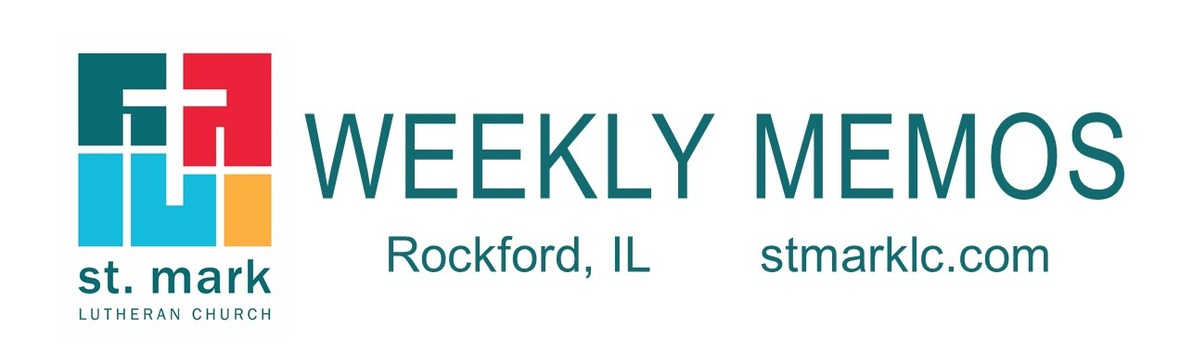/* styles */
 table div table+table+table div table{width:100%;padding:0}table div table+table+table div table img{width:96.23%;padding:0;float:none}table div table+table+table div table td{width:100%;padding:0 1.88% 18px}/* styles */## Sunday, November 10

8:00 A.M. Worship
9:30 A.M. Worship
9:30 A.M. Sunday School
11:00 A.M. Worship
3:00 P.M. The Kishwaukee Valley Concert Band - Sanctuary
7:00 P.M. 2nd Sunday at Katie's Cup

## Monday, November 11

9:00 A.M. Classic Chorale Rehearses
12:00 P.M. Belvidere Bridge Group - Cafe
4:00 P.M. Deadline for MEMOS articles to be submitted
4:30 P.M. Yoga in the Cafe
5:00 P.M. Children's Ministry
6:30 P.M. Emotions Anonymous

## Tuesday, November 12

10:00 A.M. Staff Meeting
11:00 A.M. St. Mark Duplicate Bridge

## Wednesday, November 13

9:00 A.M. Women's Bible Study
9:00 A.M. Men's Bible Study
9:00 A.M. Small Bell Group
9:30 A.M. St. Mark Ringers
12:30 P.M. Miriam Circle at Peterson Meadows
4:30 P.M. Outreach Ministry
5:30 P.M. Good News Bearers
6:00 P.M. Bible Study
6:30 P.M. Confirmation
7:00 P.M. Voices of St. Mark

## Thursday, November 14

7:30 A.M. Panera Book Study
9:00 A.M. Cherry Valley Bridge Group
9:30 A.M. Ruth Circle at St. Mark
1:00 P.M. Sew and Sews
1:00 P.M. Rebecca Circle
6:00 P.M. Diakonia
6:30 P.M. Emotions Anonymous
7:00 P.M. Praise Band Rehearses

## Friday, November 15

7:30 A.M. St. Mark Men's Breakfast at Denny's

## Saturday, November 16

8:30 A.M. Start loading the bus at St. Mark for the shopping trip to Geneva, IL

## Sunday, November 17

8:00 A.M. Worship
9:30 A.M. Worship
9:P30 A.M. Baptism of Poppy Riggins
9:30 A.M. Sunday School
11:00 A.M. Worship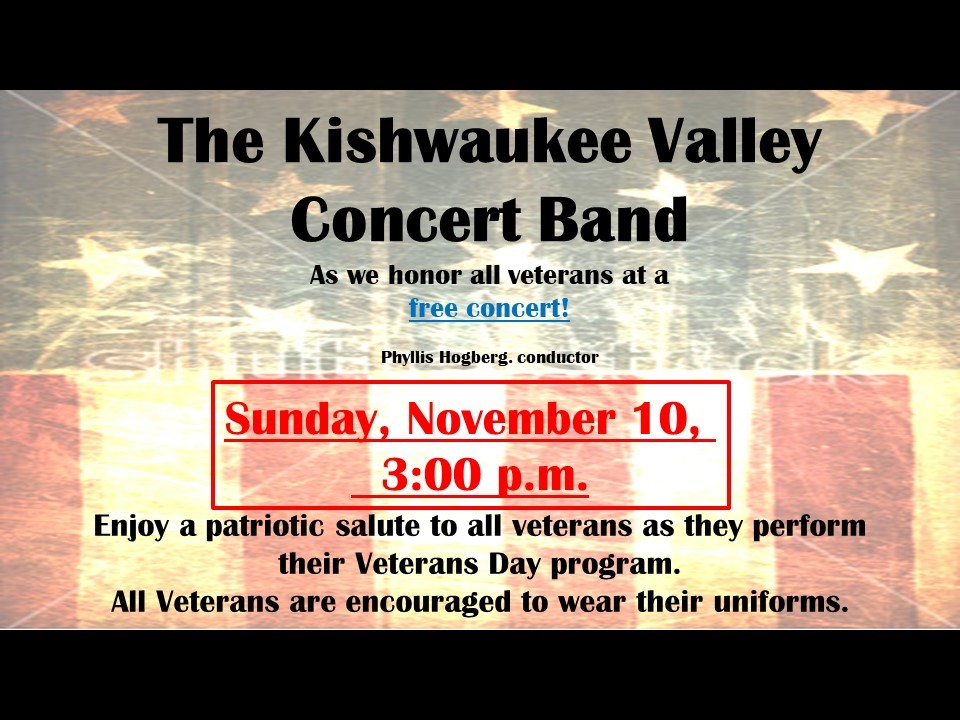table div table+table+table+table+table+table+table+table div table{width:100%;padding:0}table div table+table+table+table+table+table+table+table div table img{width:96.23%;padding:0;float:none}table div table+table+table+table+table+table+table+table div table td{width:100%;padding:0 1.88% 18px}/* styles */## News and Events

 table div table+table+table+table+table+table+table+table+table+table div table{width:100%;padding:0}table div table+table+table+table+table+table+table+table+table+table div table img{width:96.23%;padding:0;float:none}table div table+table+table+table+table+table+table+table+table+table div table td{width:100%;padding:0 1.88% 18px}/* styles */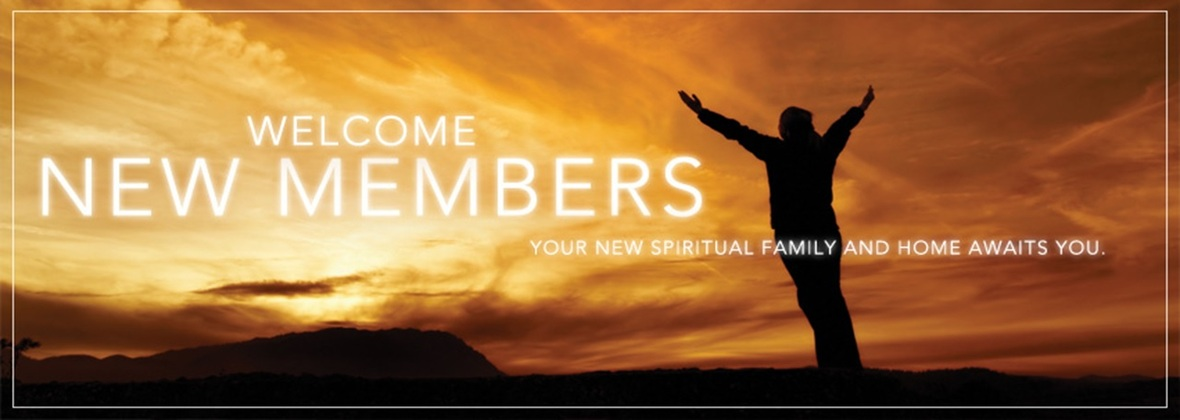Welcome New Members
St. Mark welcomed the following new
members to our congregation on Sunday, November 3: Vickie DePorter, Frank, Ramona and Savannah Drozek, Rob, Ann, Emma, and Claire James, Tim and Vicki Kenyon, and James Kirchner, Ericka Heffner and Vincent and Leonardo.

 table div table+table+table+table+table+table+table+table+table+table+table+table div table{width:100%;padding:0}table div table+table+table+table+table+table+table+table+table+table+table+table div table img{width:96.23%;padding:0;float:none}table div table+table+table+table+table+table+table+table+table+table+table+table div table td{width:100%;padding:0 1.88% 18px}/* styles */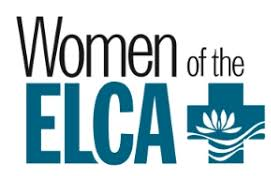Miriam Circle will meet on Wednesday, November 13 at 12:30 P.M. at Peterson Meadows. Jan Cotter and Sue Peterson are co-hosting.

The November Meeting of Ruth Circle will be held in Fellowship Hall on November 14 at 9:30 A.M. Julie Eschelman, Director of Spiritual Development, will be our guest. Come and chat with Julie and learn about our Sunday School curriculum. Inara will lead devotions and Judy Penticoff will be our hostess. Please RSVP to Judy at teachem4@comcast.net or (815) 985-3427.

 table div table+table+table+table+table+table+table+table+table+table+table+table+table+table div table{width:100%;padding:0}table div table+table+table+table+table+table+table+table+table+table+table+table+table+table div table img{width:96.23%;padding:0;float:none}table div table+table+table+table+table+table+table+table+table+table+table+table+table+table div table td{width:100%;padding:0 1.88% 18px}/* styles */Pledge Update
On October 20, we announced our 2010 Congregation Budget goal of \$827,281.00, a small increase of only \$1,481 over our 2019 budget. To date, we have received 201 pledges, totaling \$651,264.00 This amount represents 87% of the \$750,751.00 of pledged giving needed for 2020. Our pledged giving of \$750,751.00 is a key part of our total budget of \$827,281.00. This year’s stewardship campaign will continue each week until our congregation budget goal is reached. We thank you for your participation in this important expression of support and gratitude. Together, we can keep our ministry moving forward with confidence and hope.

If you have not turned in your pledge card for 2020, please do so. With everyone's assistance, we can better anticipate the full level of support from all our members for the coming year.

 table div table+table+table+table+table+table+table+table+table+table+table+table+table+table+table+table div table{width:100%;padding:0}table div table+table+table+table+table+table+table+table+table+table+table+table+table+table+table+table div table img{width:96.23%;padding:0;float:none}table div table+table+table+table+table+table+table+table+table+table+table+table+table+table+table+table div table td{width:100%;padding:0 1.88% 18px}/* styles */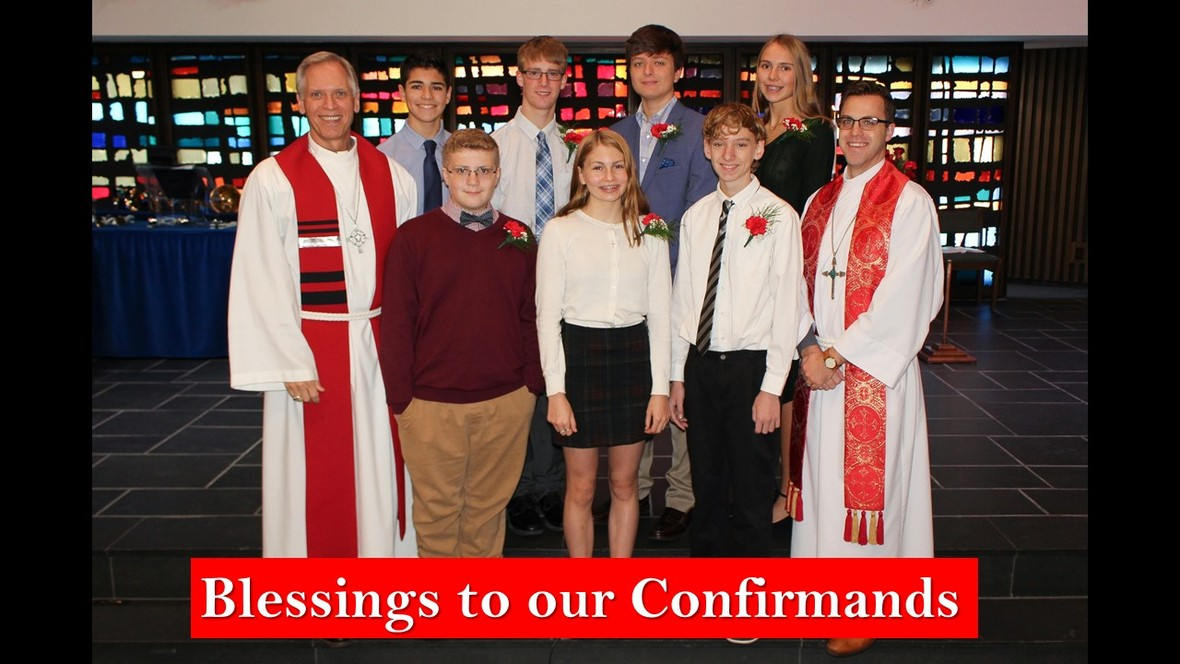/* styles */ Blessing to the following confirmands that affirmed their faith on Sunday, October 27: Ella Bjerke, Jonathan Claerbout, Alexander Crowell, Ashton Duvall, Jacob Harker, William Hatch, and Sonya McClain. Continued blessings on your faith journey.
 table div table+table+table+table+table+table+table+table+table+table+table+table+table+table+table+table+table+table+table div table{width:100%;padding:0}table div table+table+table+table+table+table+table+table+table+table+table+table+table+table+table+table+table+table+table div table img{width:96.23%;padding:0;float:none}table div table+table+table+table+table+table+table+table+table+table+table+table+table+table+table+table+table+table+table div table td{width:100%;padding:0 1.88% 18px}/* styles */Sunday is the final day to sign up for the bus trip to Geneva, Illinois.

We will load the bus at St. Mark, at 8:30 A.M. (leaving by 8:45) and return no later than 5:30 P.M. The cost is just \$35. There will be snacks and beverages on the ride home. See Mary Ann Johnson in Fellowship Hall this Sunday. Make checks payable to St. Mark or cash. Questions? E-mail Melinda@stmarklc.com 815-871-0390

 table div table+table+table+table+table+table+table+table+table+table+table+table+table+table+table+table+table+table+table+table+table div table{width:100%;padding:0}table div table+table+table+table+table+table+table+table+table+table+table+table+table+table+table+table+table+table+table+table+table div table img{width:96.23%;padding:0;float:none}table div table+table+table+table+table+table+table+table+table+table+table+table+table+table+table+table+table+table+table+table+table div table td{width:100%;padding:0 1.88% 18px}/* styles */St. Mark Mobile App!
This is different than our church directory app.
Our mobile app is designed specifically for St. Mark.

It will improve our communication with our congregation and nurture our members. A mobile app is important in the digital age. Never wonder how to get involved at St. Mark.

You will use this app for everything! You will be able to sign up for events, stay connected to your ministry group, give, send prayer requests, have an updated calendar, learn how to get involved and so much for.

We encourage you to download this app. The St. Mark app will be such a viable asset for you. It's about getting involved and staying connected.

If you have any questions, please call the office at 815-398-3557 or email Jill at Jill@stmarklc.com.

 table div table+table+table+table+table+table+table+table+table+table+table+table+table+table+table+table+table+table+table+table+table+table+table div table{width:100%;padding:0}table div table+table+table+table+table+table+table+table+table+table+table+table+table+table+table+table+table+table+table+table+table+table+table div table img{width:96.23%;padding:0;float:none}table div table+table+table+table+table+table+table+table+table+table+table+table+table+table+table+table+table+table+table+table+table+table+table div table td{width:100%;padding:0 1.88% 18px}/* styles */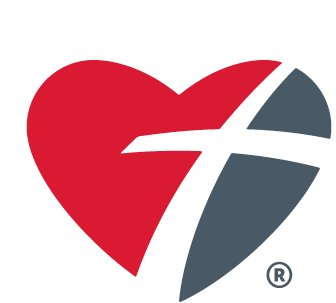Thrivent Action Team Information Session
Wednesday, Nov. 20th at 6:30 pm
Are you a Thrivent Member? Did you know that Thrivent members are eligible to receive small grants in order to do service projects and learning events in the community? Find out more at this hour-long session on Action Teams, presented by Steve Steiner and Lee Strauman from Thrivent Financial. A pizza dinner will be provided. RSVP to Pastor Chad if you plan to attend.prmckenna@stmarklc.com.

 table div table+table+table+table+table+table+table+table+table+table+table+table+table+table+table+table+table+table+table+table+table+table+table+table+table div table{width:100%;padding:0}table div table+table+table+table+table+table+table+table+table+table+table+table+table+table+table+table+table+table+table+table+table+table+table+table+table div table img{width:96.23%;padding:0;float:none}table div table+table+table+table+table+table+table+table+table+table+table+table+table+table+table+table+table+table+table+table+table+table+table+table+table div table td{width:100%;padding:0 1.88% 18px}/* styles */Yoga is Happening at St. Mark!
Join us on November 11 and December 2 and 9 at 4:30 p.m. in the St. Mark Café space for Yoga. Dress comfortable, if you have a yoga mat bring it…otherwise, we have mats you may borrow. Also, bring a water bottle. Betsy Hornick is our yoga instructor and she is doing a great job of leading us through the workout.

 table div table+table+table+table+table+table+table+table+table+table+table+table+table+table+table+table+table+table+table+table+table+table+table+table+table+table+table div table{width:100%;padding:0}table div table+table+table+table+table+table+table+table+table+table+table+table+table+table+table+table+table+table+table+table+table+table+table+table+table+table+table div table img{width:96.23%;padding:0;float:none}table div table+table+table+table+table+table+table+table+table+table+table+table+table+table+table+table+table+table+table+table+table+table+table+table+table+table+table div table td{width:100%;padding:0 1.88% 18px}/* styles */St. Mark Prayer Chain
Would you like to be a part of the St. Mark prayer chain? Prayer requests come into the church office weekly. As we receive prayer requests we would send out an e-mail and ask that you pray daily for the persons mentioned in the requests. All requests are to remain confidential. Please e-mail melinda@stmarklc.com if you would like to participate in the prayer chain. “Be anxious for nothing, but in everything by prayer and supplication, with thanksgiving, let your requests be made known to God.” Colossians 4:2.

 table div table+table+table+table+table+table+table+table+table+table+table+table+table+table+table+table+table+table+table+table+table+table+table+table+table+table+table+table+table div table{width:100%;padding:0}table div table+table+table+table+table+table+table+table+table+table+table+table+table+table+table+table+table+table+table+table+table+table+table+table+table+table+table+table+table div table img{width:96.23%;padding:0;float:none}table div table+table+table+table+table+table+table+table+table+table+table+table+table+table+table+table+table+table+table+table+table+table+table+table+table+table+table+table+table div table td{width:100%;padding:0 1.88% 18px}/* styles */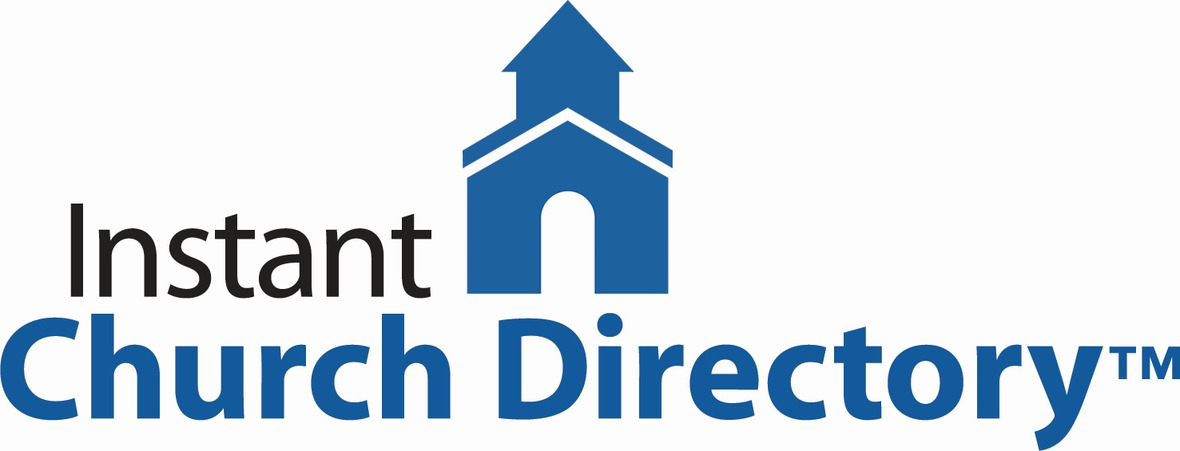Instant Church Directory
If you have not submitted a family photo for the online directory, please follow the links below.
It is simple and takes only a few minutes to upload your photo and then download the Instant Church Directory App. You will always have updated members' information at your fingertips.

Instant Church Directory

Once you have downloaded the app and created an account, you are not able to change or update your information, the system administrator is the only one to change and update the information. If something is incorrect, please contact the church office at 815-398-3557 or email jill@stmarklc.com.

Mobile App Login Instructions for Android & Kindle Devices

We also have a form for you to complete to make sure we have the most current information for the directory. There are paper forms at church or click on the link to Church Directory Form

 table div table+table+table+table+table+table+table+table+table+table+table+table+table+table+table+table+table+table+table+table+table+table+table+table+table+table+table+table+table+table+table div table{width:100%;padding:0}table div table+table+table+table+table+table+table+table+table+table+table+table+table+table+table+table+table+table+table+table+table+table+table+table+table+table+table+table+table+table+table div table img{width:96.23%;padding:0;float:none}table div table+table+table+table+table+table+table+table+table+table+table+table+table+table+table+table+table+table+table+table+table+table+table+table+table+table+table+table+table+table+table div table td{width:100%;padding:0 1.88% 18px}/* styles */Do you like to “wrap” gifts?
We need a few helpers who like to wrap, on Sunday, November 24, between services.
We have a special holiday project that involves wrapping some boxes. Sign-up in Fellowship Hall if you can help. Watch for more information on this special holiday project!

 table div table+table+table+table+table+table+table+table+table+table+table+table+table+table+table+table+table+table+table+table+table+table+table+table+table+table+table+table+table+table+table+table+table div table{width:100%;padding:0}table div table+table+table+table+table+table+table+table+table+table+table+table+table+table+table+table+table+table+table+table+table+table+table+table+table+table+table+table+table+table+table+table+table div table img{width:96.23%;padding:0;float:none}table div table+table+table+table+table+table+table+table+table+table+table+table+table+table+table+table+table+table+table+table+table+table+table+table+table+table+table+table+table+table+table+table+table div table td{width:100%;padding:0 1.88% 18px}/* styles */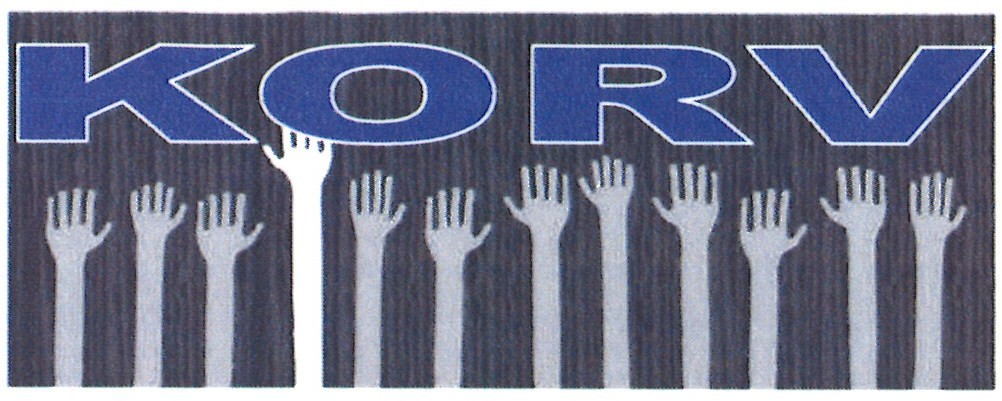Who is ready for the annual korv sale? Rockford Area Lutheran Ministries is gearing up for korv 2019 and we are ready to accept sales and volunteers.

RALM is celebrating making the Tabor traditional Swedish korv. Telling our Swedish heritage through 1200 pounds of sausage is a newer adventure relating with our community.

Korv making days this year are December 3rd, 4th and 5th with pick-up on December 4th and 5th.

To volunteer and place an order email ralmmail@aol.com, call 815-962-4279. Click this link rockthegood.com for the order form.

Same Tabor korv, but now through Rockford Area Lutheran Ministries and prepared at Zion.

 table div table+table+table+table+table+table+table+table+table+table+table+table+table+table+table+table+table+table+table+table+table+table+table+table+table+table+table+table+table+table+table+table+table+table+table div table{width:100%;padding:0}table div table+table+table+table+table+table+table+table+table+table+table+table+table+table+table+table+table+table+table+table+table+table+table+table+table+table+table+table+table+table+table+table+table+table+table div table img{width:96.23%;padding:0;float:none}table div table+table+table+table+table+table+table+table+table+table+table+table+table+table+table+table+table+table+table+table+table+table+table+table+table+table+table+table+table+table+table+table+table+table+table div table td{width:100%;padding:0 1.88% 18px}/* styles */## Youth Ministry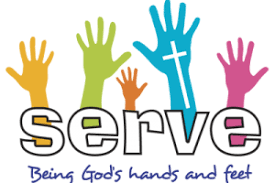Service Learning Camps Summer 2020!
“Where the learning is just as important as the service.” If you will be entering high school in the fall of 2020 or are already in high school then this is for you! We will be traveling to Toledo, Ohio July 12-17th, 2020. There will be service projects and a day of sightseeing.

We need to get a commitment by January 1, if you are interested. The cost is \$350, with a deposit of \$50. Due January 1, 2020.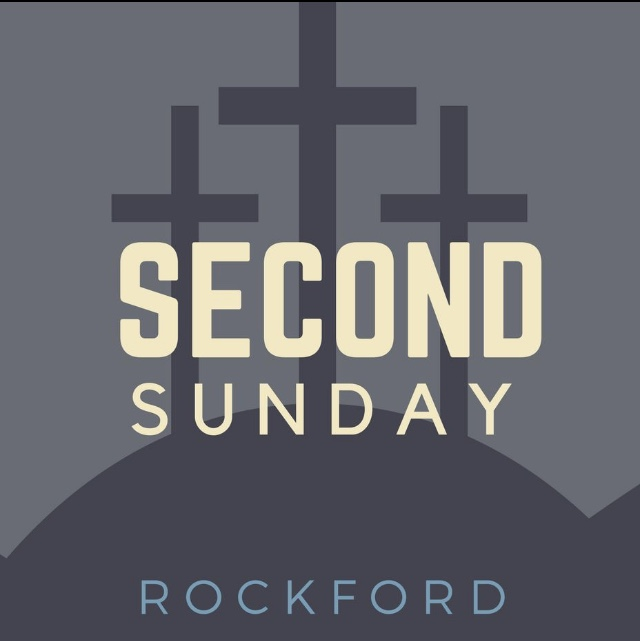High School Youth, Second Sunday is happening this Sunday, November 10, at 7:00 P.M. Join us at Katie’s Cup or see Melinda Alekna if you would like a ride. (815-871-0390.) This month we will talk about “thankfulness” and we will also be taste testing some holiday food! Join us for food, friends, free coffee drinks, and fellowship. And also youth-led worship! Hope to see you on Sunday!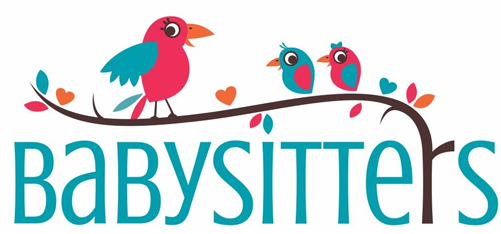Middle School and High School Youth “babysitter” list.
On occasion, parents will ask for the name of one of our youth who babysits.
Would you like to be on that list? You don’t have to be a certified babysitter, but if you take a class or course let me know. Please e-mail melinda@stmarklc.com or text me at 815-871-0390. Need more information see Melinda Alekna.

 table div table+table+table+table+table+table+table+table+table+table+table+table+table+table+table+table+table+table+table+table+table+table+table+table+table+table+table+table+table+table+table+table+table+table+table+table+table+table+table+table div table{width:100%;padding:0}table div table+table+table+table+table+table+table+table+table+table+table+table+table+table+table+table+table+table+table+table+table+table+table+table+table+table+table+table+table+table+table+table+table+table+table+table+table+table+table+table div table img{width:96.23%;padding:0;float:none}table div table+table+table+table+table+table+table+table+table+table+table+table+table+table+table+table+table+table+table+table+table+table+table+table+table+table+table+table+table+table+table+table+table+table+table+table+table+table+table+table div table td{width:100%;padding:0 1.88% 18px}/* styles */## Primetimers Ministry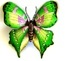November 10 Sunday at 3:00 pm meet at St. Mark. As a tribute to our Veterans, the Kishwaukee Band will play our favorite patriotic hymns and songs. Afterward, let us drive over to the Olive Garden to enjoy dinner together. Please sign up on Sunday morning or call Mary Ann Johnson at 815-397-5882.

November 21, Thursday, in Fellowship Hall at noon partake of a slice of pizza, relax and enjoy a powerpoint lecture.

Everyone probably knows something about Leonardo da Vinci. This year museums and fashion houses, scientists and inventors around the world had been celebrating the life of Leonardo da Vinci; it has been 500 years since his death on May 12, 1519. He is known as the inventor of shoes to walk on water and submarines. His dissections of the human body proceed with the advances in science and many medical fields by centuries. Join art historian, Laura C. Johnson for a deeper look into this quintessential Renaissance man, as she shows how his designs are used by fashion designers in Paris; how he influenced the way we roast poultry to this day. Other favorite subjects such as napkins, the tongue of a woodpecker, and the extraordinary story of a painting contest between da Vinci and Michelangelo. She will also reveal why actor Leonardo di Caprio was named Leonardo!

All are welcome: please sign up on Sunday so we may plan according or call Mary Ann Johnson 815-397-5882.

 table div table+table+table+table+table+table+table+table+table+table+table+table+table+table+table+table+table+table+table+table+table+table+table+table+table+table+table+table+table+table+table+table+table+table+table+table+table+table+table+table+table+table+table div table{width:100%;padding:0}table div table+table+table+table+table+table+table+table+table+table+table+table+table+table+table+table+table+table+table+table+table+table+table+table+table+table+table+table+table+table+table+table+table+table+table+table+table+table+table+table+table+table+table div table img{width:96.23%;padding:0;float:none}table div table+table+table+table+table+table+table+table+table+table+table+table+table+table+table+table+table+table+table+table+table+table+table+table+table+table+table+table+table+table+table+table+table+table+table+table+table+table+table+table+table+table+table div table td{width:100%;padding:0 1.88% 18px}/* styles */## Outreach MinistryRemember a foster child this Christmas. The Outreach Committee is asking you to take an angel tag from the display this November and shop for a gift for a foster child. “Adopt-an Angel” is a joint effort of the agencies in Winnebago and Boone Counties to see that every child receives a Christmas present. We are working with the Lutheran Social Services of Illinois. This agency alone has over 350 children in the Foster Care Alliance.

The tags will provide 3 gift ideas requested by the child. Some of the teenage children may request gift cards. They received very little personal spending money each month, so many look forward to being able to go shopping. The suggested gift value is \$20 per child. This information was provided by LSSI.

Wrapped gifts will be due back at church before the end of November. Keep an eye out for the Angel Tree!

 table div table+table+table+table+table+table+table+table+table+table+table+table+table+table+table+table+table+table+table+table+table+table+table+table+table+table+table+table+table+table+table+table+table+table+table+table+table+table+table+table+table+table+table+table+table+table+table div table{width:100%;padding:0}table div table+table+table+table+table+table+table+table+table+table+table+table+table+table+table+table+table+table+table+table+table+table+table+table+table+table+table+table+table+table+table+table+table+table+table+table+table+table+table+table+table+table+table+table+table+table+table div table img{width:96.23%;padding:0;float:none}table div table+table+table+table+table+table+table+table+table+table+table+table+table+table+table+table+table+table+table+table+table+table+table+table+table+table+table+table+table+table+table+table+table+table+table+table+table+table+table+table+table+table+table+table+table+table+table div table td{width:100%;padding:0 1.88% 18px}/* styles */## Barnabas Ministry

 table div table+table+table+table+table+table+table+table+table+table+table+table+table+table+table+table+table+table+table+table+table+table+table+table+table+table+table+table+table+table+table+table+table+table+table+table+table+table+table+table+table+table+table+table+table+table+table+table+table div table,table.module-48{width:3.77%;float:left;padding:0}table div table+table+table+table+table+table+table+table+table+table+table+table+table+table+table+table+table+table+table+table+table+table+table+table+table+table+table+table+table+table+table+table+table+table+table+table+table+table+table+table+table+table+table+table+table+table+table+table+table div table a{border:0 none;text-decoration:none}table div table+table+table+table+table+table+table+table+table+table+table+table+table+table+table+table+table+table+table+table+table+table+table+table+table+table+table+table+table+table+table+table+table+table+table+table+table+table+table+table+table+table+table+table+table+table+table+table+table div table img{width:100%!important;border:0 none;text-decoration:none}table div table+table+table+table+table+table+table+table+table+table+table+table+table+table+table+table+table+table+table+table+table+table+table+table+table+table+table+table+table+table+table+table+table+table+table+table+table+table+table+table+table+table+table+table+table+table+table+table+table div table td{width:100%;padding:0 20px 20px 0}/* styles */ Dear friend, today I was talking to a man who recently lost his mother. She was in a nursing home. His father is currently in a nursing home, with dementia. As we talked, I thanked him for his outstanding care of his parents and the regular schedule he has established for himself to visit his father now. I said that so many elders are so lonely and neglected. He so affirmed that! He said elders reached out to him constantly in both nursing homes, and he felt overwhelmed by their needs. We have wonderful people at St. Mark just as faithful as he is. The Barnabas ministers' one mission is to encourage by listening. We will be meeting on Thursday, November 21 at 2 p.m. in the cafe. Would you sit in on our meeting? More information? Just call me at 815-342-6064. Blessed day, Pastoral care Pastor Chuck Olson
 table div table+table+table+table+table+table+table+table+table+table+table+table+table+table+table+table+table+table+table+table+table+table+table+table+table+table+table+table+table+table+table+table+table+table+table+table+table+table+table+table+table+table+table+table+table+table+table+table+table+table div table{width:100%;padding:0}table div table+table+table+table+table+table+table+table+table+table+table+table+table+table+table+table+table+table+table+table+table+table+table+table+table+table+table+table+table+table+table+table+table+table+table+table+table+table+table+table+table+table+table+table+table+table+table+table+table+table div table img{width:96.23%;padding:0;float:none}table div table+table+table+table+table+table+table+table+table+table+table+table+table+table+table+table+table+table+table+table+table+table+table+table+table+table+table+table+table+table+table+table+table+table+table+table+table+table+table+table+table+table+table+table+table+table+table+table+table+table div table td{width:100%;padding:0 1.88% 18px}/* styles */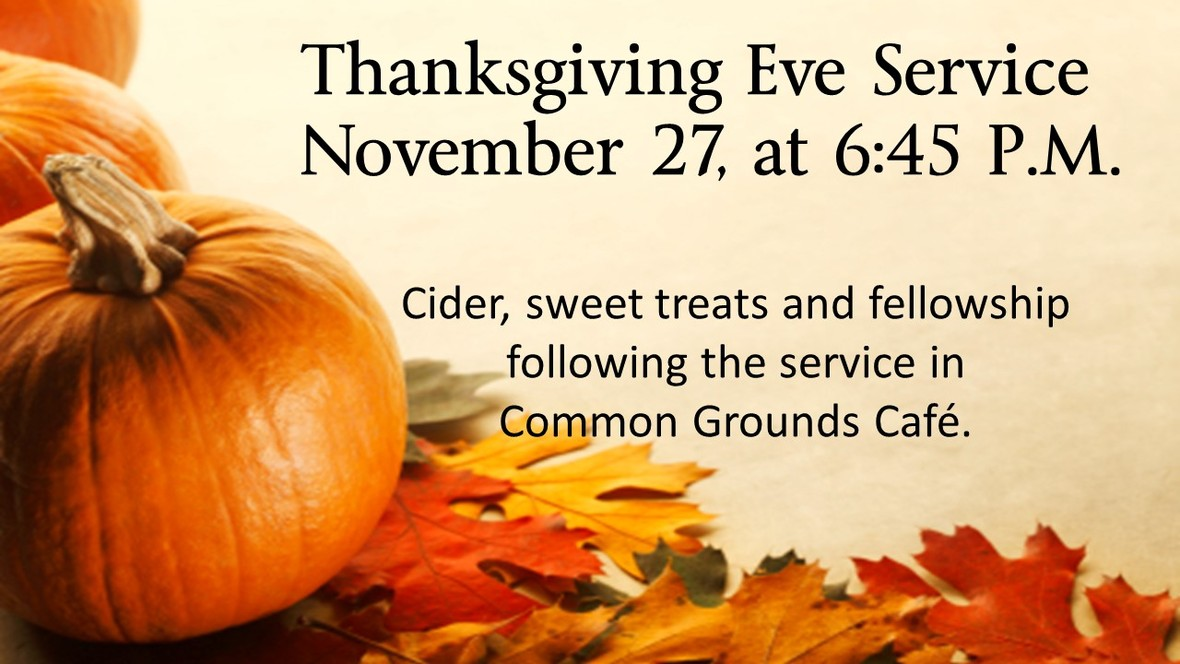table div table+table+table+table+table+table+table+table+table+table+table+table+table+table+table+table+table+table+table+table+table+table+table+table+table+table+table+table+table+table+table+table+table+table+table+table+table+table+table+table+table+table+table+table+table+table+table+table+table+table+table+table div table{width:100%;padding:0}table div table+table+table+table+table+table+table+table+table+table+table+table+table+table+table+table+table+table+table+table+table+table+table+table+table+table+table+table+table+table+table+table+table+table+table+table+table+table+table+table+table+table+table+table+table+table+table+table+table+table+table+table div table img{width:96.23%;padding:0;float:none}table div table+table+table+table+table+table+table+table+table+table+table+table+table+table+table+table+table+table+table+table+table+table+table+table+table+table+table+table+table+table+table+table+table+table+table+table+table+table+table+table+table+table+table+table+table+table+table+table+table+table+table+table div table td{width:100%;padding:0 1.88% 18px}/* styles */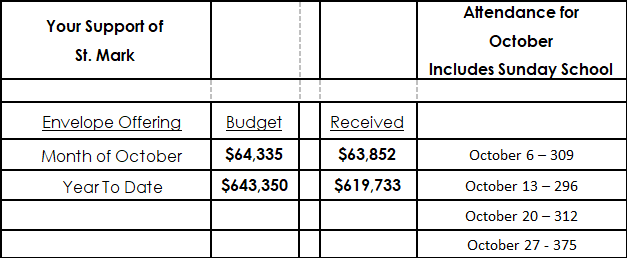table div table+table+table+table+table+table+table+table+table+table+table+table+table+table+table+table+table+table+table+table+table+table+table+table+table+table+table+table+table+table+table+table+table+table+table+table+table+table+table+table+table+table+table+table+table+table+table+table+table+table+table+table+table+table div table{width:100%;padding:0}table div table+table+table+table+table+table+table+table+table+table+table+table+table+table+table+table+table+table+table+table+table+table+table+table+table+table+table+table+table+table+table+table+table+table+table+table+table+table+table+table+table+table+table+table+table+table+table+table+table+table+table+table+table+table div table img{width:96.23%;padding:0;float:none}table div table+table+table+table+table+table+table+table+table+table+table+table+table+table+table+table+table+table+table+table+table+table+table+table+table+table+table+table+table+table+table+table+table+table+table+table+table+table+table+table+table+table+table+table+table+table+table+table+table+table+table+table+table+table div table td{width:100%;padding:0 1.88% 18px}/* styles */## Our St. Mark members:

Bette Patterson, Jason Carlson, Steve Carter, Beverly Wright, Peggy Bargren, Jim Tooley, Jerry Cazaniga, David Young, Keith Haggestad.

## Family and friends of St. Mark members:

Jessica Salter, Bill Ganschow, Tim Babcock, Polly Young, Eva Love Sherbondy, Matthew and David Lundine, Jennifer Peterson, Lynn Reed, Dale Tallmadge, Pastor Jane McChesney, Earl Karau, Ann Eck, Clay Heinrich, Denise Nichols, Mary Graham, Barbara Heckart, Kim Menke, Norma Comstock, Ethan Whitney, Carolyn King, Katie Butera, Ashton Henley, Nathan Gyllin.

## Those serving in the military and their families:

Tristan Davenport, Scot Hornick, Bailey Panjkovich, Xander Gehrke, Jim Tammen, Brandon Ballenger, Taylor Eversole, Dean Barron.

 table div table+table+table+table+table+table+table+table+table+table+table+table+table+table+table+table+table+table+table+table+table+table+table+table+table+table+table+table+table+table+table+table+table+table+table+table+table+table+table+table+table+table+table+table+table+table+table+table+table+table+table+table+table+table+table+table+table div table{width:100%;padding:0}table div table+table+table+table+table+table+table+table+table+table+table+table+table+table+table+table+table+table+table+table+table+table+table+table+table+table+table+table+table+table+table+table+table+table+table+table+table+table+table+table+table+table+table+table+table+table+table+table+table+table+table+table+table+table+table+table+table div table img{width:96.23%;padding:0;float:none}table div table+table+table+table+table+table+table+table+table+table+table+table+table+table+table+table+table+table+table+table+table+table+table+table+table+table+table+table+table+table+table+table+table+table+table+table+table+table+table+table+table+table+table+table+table+table+table+table+table+table+table+table+table+table+table+table+table div table td{width:100%;padding:0 1.88% 18px}/* styles *//* styles */ Office Hours Monday - Friday, 8:00 A.M. - 4:00 P.M. Telephone: 815-398-3557 Publications St. Mark Memos, the weekly e-newsletter, is distributed each Thursday. The deadline for submission is Monday by 4:00 P.M. Please click to send submissions. Sermons Online Miss a Sunday at St. Mark? Please visit our website and watch the sermons online. Please click to watch.